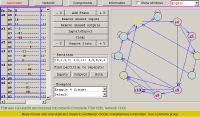Start the applet

INTRODUCTION

Applet demonstrates additive decomposition, wherein the graph of the FSM is partitioned into node disjoint subsets. The network of interacting sub-FSMs corresponds to a given partition on the set of states of source FSM. The network of FSMs consists of components working alternatively in time, i.e. all components except one are suspended in one of extra state (the “wait” state). This property gives opportunity to apply sleep mode operation (dynamic power management) for saving power consumption.

SHORT THEORETICAL BASICS

Prototype FSM model

Formally, FSM is a quintuple < S, X, Y,,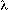> where

• S = { s1, … , sn } is a set of states;
• X = { x1, … , xl } is a set of primary input variables;
• Y = { y1, … , ym } is a set of primary output variables;
•: D()S is a multiple valued next state function with
domain D () ={0, 1}
l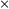S and codomain S ;
{0, 1} represents a set of values (symbols) each input variable xi may assume;
•: D()R() is an output function with
domain D() = {0, 1} lS and codomain R() ={0, 1}m ;
{0, 1} is a set of values each output variable yi may assume.

Example Prototype FSM

 S= { s1 , s2 , … , s8 } X= { x1, x2 , … , x8 } Y= { y1 , y2 , … , y14 }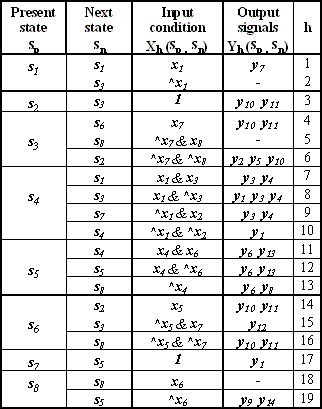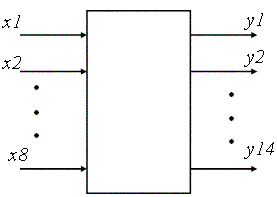FSM descriptionDecomposition procedures

• Generation of the initial partition
• Definition of states in component FSMs
• Definition of the set of external input variables of component FSM
• Generation of the set of the set of internal (additional) input variables
• Definition of the set of output variables of component FSMs
• Generation of transition and output functions of component FSMs
• Realization of FSM network.

Partitions

The set of states of (component) sub-FSM

Define set of states in component automata as: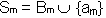where:
Bm- is the block of the partition,
am - is the additional state that exists in each component automata.

Let define states of component automata in our example:

S={ s1 , s2 , … , s8 }={ { s1, s4, s7 }, { s2 , s3, s6 }, { s5 , s8 } }

S1 = { s1, s4, s7, a1 }
S2 = { s2, s3, s6, a2 }
S3 = { s5, s8, a3 }

Set of external input variables

 X(Bm) is the set of external input variables at all transitions from the states of block Bm in the transition table of the prototype FSM A: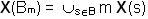In our example: X(B1) = { x1, x2, x3 } X(B2) = { x5, x7, x8 } X(B3) = { x4, x6 }={ { s1, s4, s7 }, { s2 , s3, s6 }, { s5 , s8 } }Set of external output variables
 Y(Bm) is the set of external output variables at all transitions from the states of block Bm in the transition table of the FSM A:In our example: Y(B1) = { y3, y4, y7 } Y(B2) = { y2, y5, y10, y11, y12 } Y(B3) = { y6, y8, y9, y13, y14 }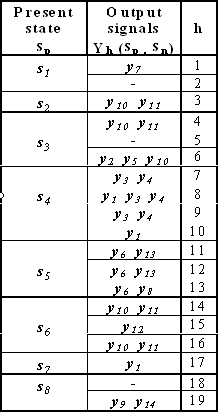Example FSM parameters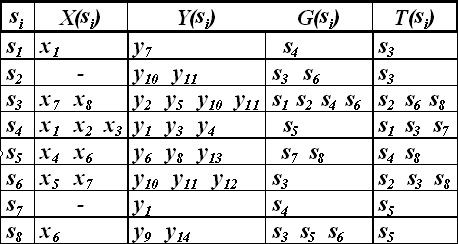Component FSMs B2 and B3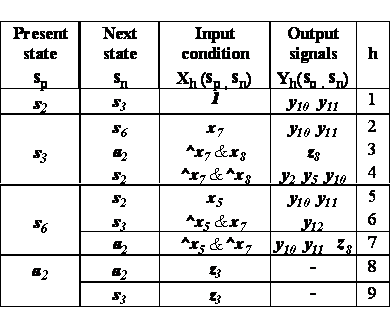FSM NetworkAdditional theory is available here or here

APPLET MANUAL

LIST OF PUBLICATIONS:

WARNING: This page contains links to PDF files of articles that may be covered by copyright. You may browse the articles at your convenience. (in the same spirit as you may read a journal or a proceeding article in a public library). Retrieving, copying, or distributing these files, however, may violate the copyright protection law. We recommend that the user abides international law in accessing this directory.

Last update: 3 August, 2004